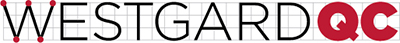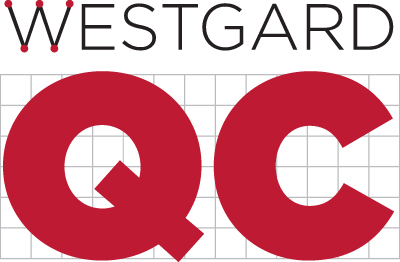QC Frequency Calculator
Analyst
Date
Analyzer
Test
Units
Levels of Controls Average
Concentration
Quality Requirement, TEa
Bias observed
Precision observed
Calculated Sigma-metric
Patient Risk Sigma
Applied Patient Risk Factor
Maximum Run Size
 Candidate SQC Procedures Run Size 1 Run Size 2 Run Size 3 Run Size Av 1:3s/2of3:2s/3:1s/6:X N=6 (Pfr=0.07) 1:2.5s, N=6 (Pfr=0.06) 1:3s/2of3:2s/3:1s N=3 (Pfr=0.02) 1:2.5s, N=3 (Pfr=0.03) 1:3s N=3 (Pfr=0.01) 1:2s, N=1 (Pfr=0.05) 1:2.5s, N=1 (Pfr=0.01) 1:3s, N=1 (Pfr=0.00)